# KSEEB Solutions for Class 8 Maths Chapter 9 Commercial Arithmetic Ex 9.5

In this chapter, we provide KSEEB SSLC Class 8 Maths Chapter 9 Commercial Arithmetic Ex 9.5 for English medium students, Which will very helpful for every student in their exams. Students can download the latest KSEEB SSLC Class 8 Maths Chapter 9 Commercial Arithmetic Ex 9.5 pdf, free KSEEB SSLC Class 8 Maths Chapter 9 Commercial Arithmetic Ex 9.5 pdf download. Now you will get step by step solution to each question.

### Karnataka State Syllabus Class 8 Maths Chapter 9 Commercial Arithmetic Ex 9.5

Question 1.
Find the simple interest on Rs. 2500 for 4 years at 6 14 % per annum.
P = Rs. 2500
T = 4 years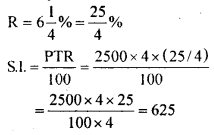Simple interest is Rs. 625.

Question 2.
Find the simple interest on Rs. 3500 at the rate of 214% per annum for 165 days.
P= Rs. 3500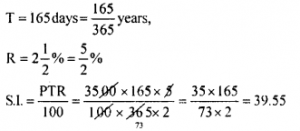Simple interest = Rs. 39.55.

Question 3.
In what period will Rs. 5200 amounts to Rs. 7,384 at 12% per annum simple interest?
P = Rs. 5200
Amount = Rs. 7384
R = 12%
T = ?
Simple interest = Amount – principal = 7384 – 5200 = Rs. 2184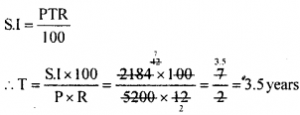If amounts to Rs. 7,384 in 3.5 years

Question 4.
Ramya borrowed a loan from a bank for buying a computer. After 4 years she paid Rs. 26,640 and settled the account. If the rate of interest is 12% per annum what was the sum she borrowed?
T = 4 years
R = 12%
After 4 years she paid Rs. 26,640.
This is nothing but amount.
Let the principal borrowed be R Amount = principal + S.I.
∴ Simple interest = Amount – principal S.I. = 26,640 – P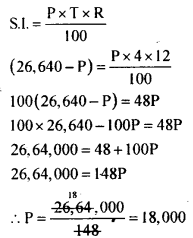The principal borrowed – Rs. 18,000

Question 5.
A sum of money triples itself in 8 years. Find the rate of interest.
Let the principal be x. It amounts to 3x in 8 years.
Amount = principal + simple interest
∴ Simple interest = Amount – principal = 3x – x = 2x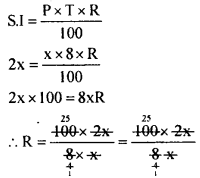R = 25%

All Chapter KSEEB Solutions For Class 8 maths

—————————————————————————–

All Subject KSEEB Solutions For Class 8

*************************************************

I think you got complete solutions for this chapter. If You have any queries regarding this chapter, please comment on the below section our subject teacher will answer you. We tried our best to give complete solutions so you got good marks in your exam.

If these solutions have helped you, you can also share kseebsolutionsfor.com to your friends.

Best of Luck!!